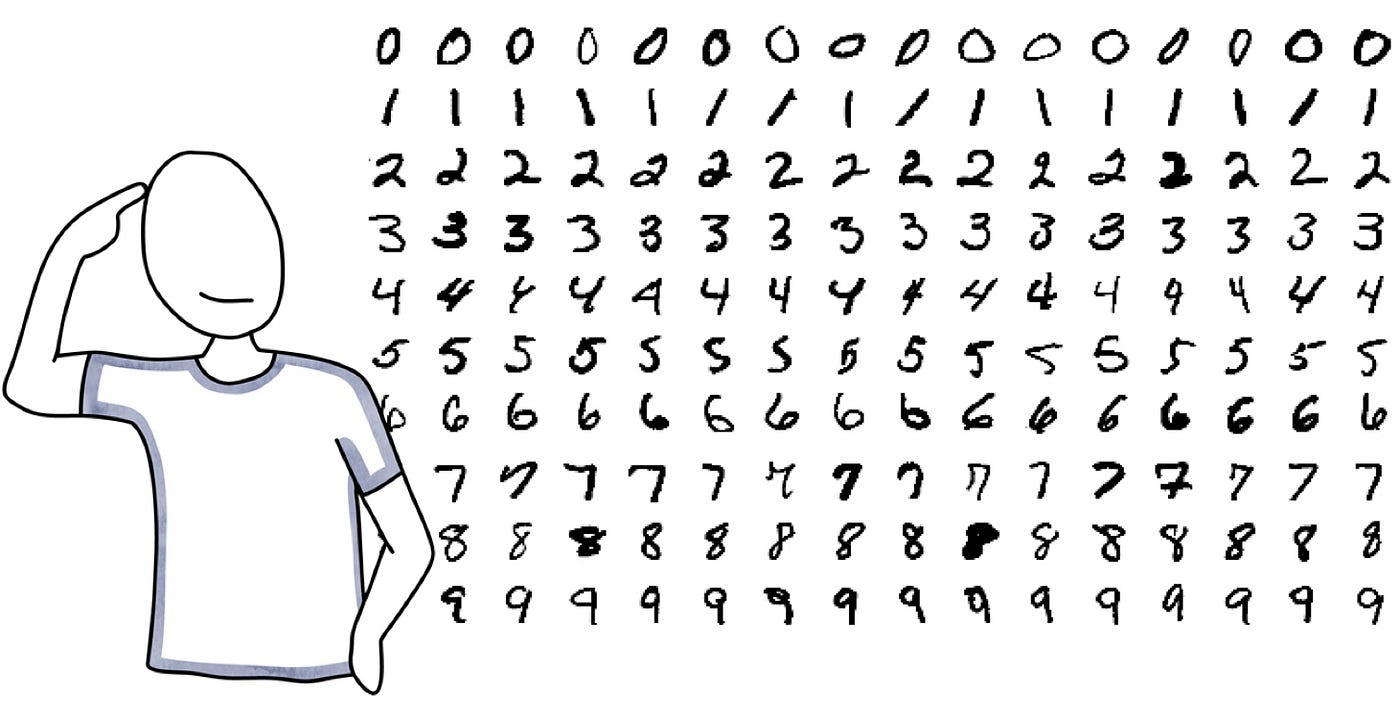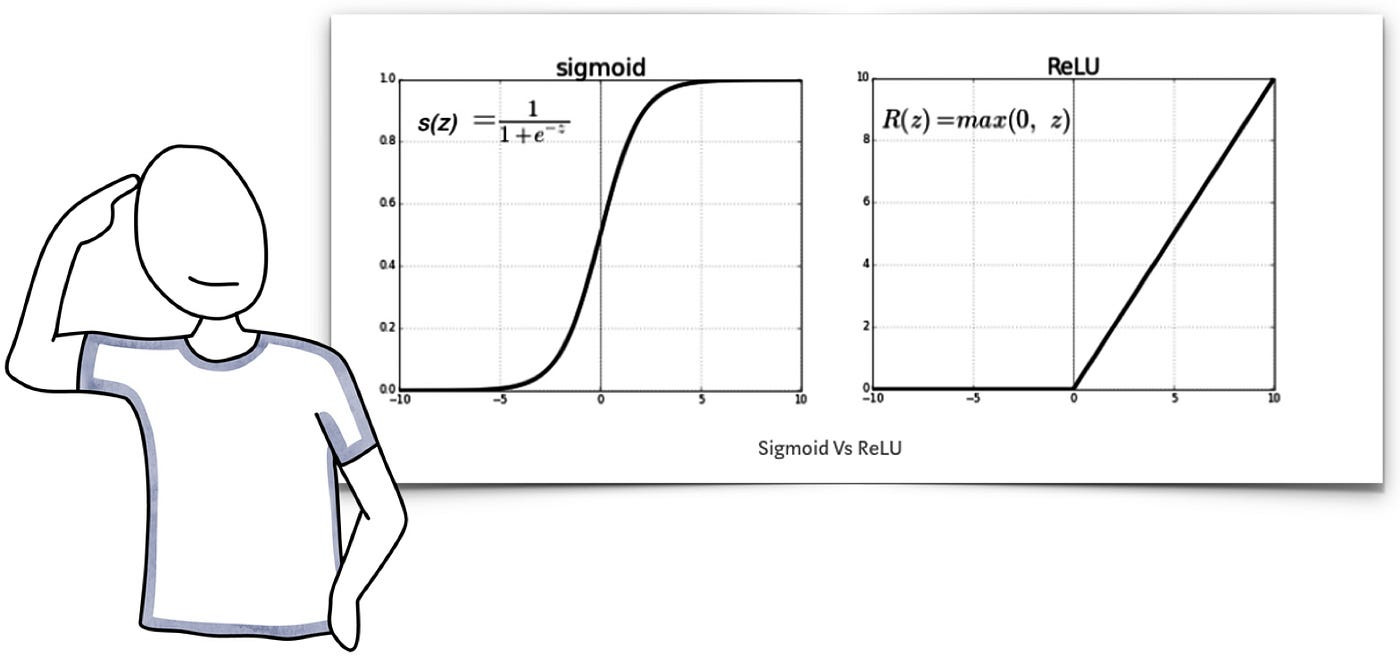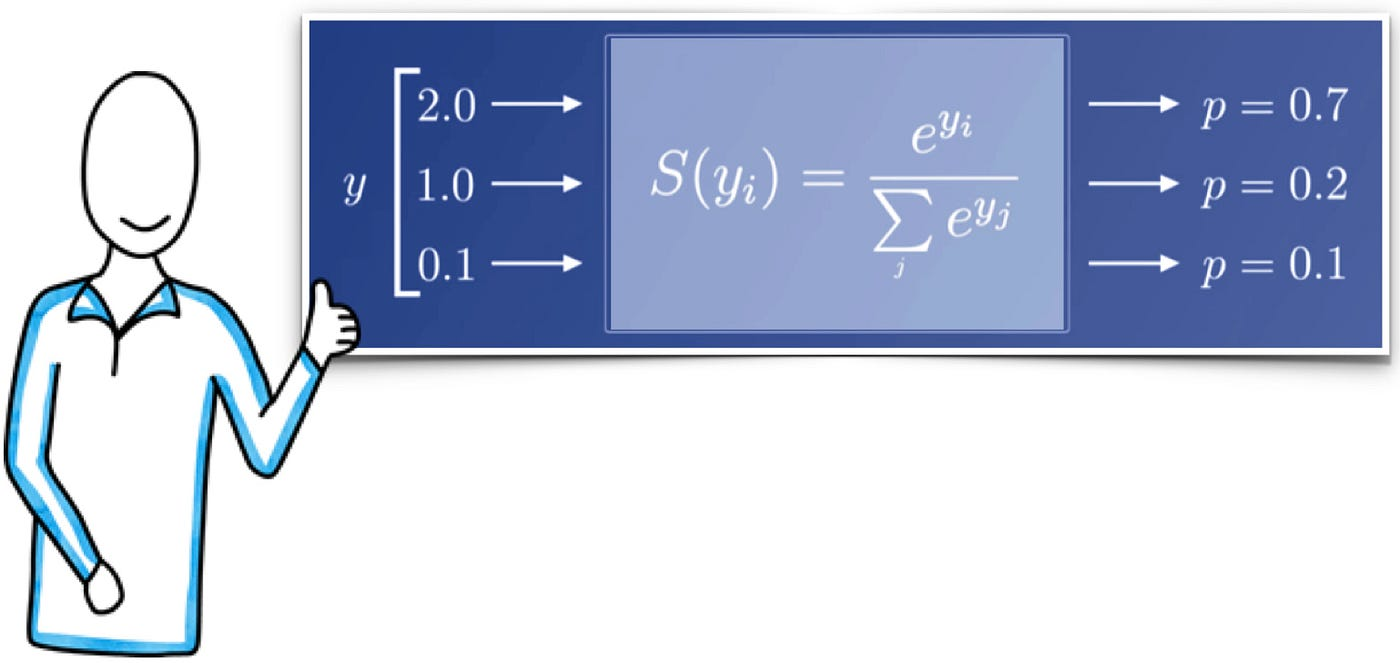# DeepLearning4J: Simple Image Classification

Let’s review how to implement image classification with neural networks using the Deeplearning4j library. If you are new to neural networks, you would like to read my introductory story, What is a Neural Network?. And, if you are not familiar with the DeepLearning4J library, I could recommend looking at my story, DeepLearning4J: Getting Started.

Using neural networks for image classification is a very straightforward process. We need a dataset with multiple images that we can use to train our neural network. We will use as inputs every single pixel in every picture. And, we expect to get as output the category that we are looking forward to recognizing.

Let us work on a simple project: a model to recognize handwriting numbers 0 to 9. We are going to do the following:

1. Get and Load a dataset
2. Create, train, and evaluate a model
3. Deploy the model in an application

I will split this article into two parts. In this part, I will cover the dataset and the model. Later we can review the deployment of our model.

# 1. The MNIST dataset

We aim to create a neural network to recognize handwriting numbers 0 to 9. Thus, we need images with tons of handwritten digits. One of the most famous datasets containing handwritten digits is the MNIST dataset, consisting of samples, as shown in Figure 1. It is an extensive database of handwritten digits comprised of 70,000 images. The numerals were written by high school students and the United States Census Bureau employees. Each number is stored as an anti-aliased image in black and white and is normalized to fit into a 28x28 pixel bounding box.Figure 1. Sample images from the MNIST dataset; each digit is stored as a 28x28 pixels image

As a reference, according to the MNIST website, a one-layer neural network (trained with this dataset) could achieve an error rate of 12% (pretty bad). In comparison, a deep convolutional neural network could achieve an error rate below 0.25%.

DeepLearning4j comes with out-of-the-box dataset iterators for standard datasets, including the MNIST dataset. The class MnistDataSetIterator allows us to load this common dataset. The constructor for MnistDataSetIterator receives three parameters:

• The batch size, i.e., the number of training samples to work through before comparing the expected output and calculating the error.
• The total number of samples in the dataset.
• A flag to indicate whether the dataset should be binarized (images considered in black & white without shades of gray) or not.

Let us use a batch size of 100 and ask for the dataset to be considered black & white images. Moreover, let us load 60,000 images for training and 10,000 images for testing, as follows:

Pretty simple. No need to worry about the technical details of handling a data structure with thousands of 28 x 28 images.

# 3. Building a First Model

Let’s start with a basic single hidden layer neural network. Later, we could improve this initial approach. A single hidden layer neural network for solving this problem could be as follows:

• Each pixel in the images became an input; therefore, we have 28 x 28 = 784 inputs.
• Each of the digits we want to predict becomes an output; therefore, we have ten neurons in the output layer.
• Finally, the number of hidden neurons in a single hidden layer model is suggested to be either: between the size of the input and the output layers; 2/3 the size of the input layer, plus the size of the output layer. ; or less than twice the size of the input layer. These three rules provide a starting point for you to consider. Ultimately, selecting an architecture for your neural network will come down to trial and error. Let’s use 1000 neurons in the hidden layer.

Now, we can create a single hidden layer neural network using the classes MultiLayerConfiguration and MultiLayerNetwork, emulating what we did in Getting Started with DeepLearning4J, as follows:

The class MultiLayerConfiguration is the one doing the magic:

• 784 inputs connected all to 1000 neurons in an intermediate layer using SIGMOID as activation function
• 1000 neurons in an intermediate layer connected to 10 neurons in the output layer using SIGMOID as activation and MSE as loss functions.

That MultiLayerConfiguration object is used as input for the MultiLayerNetwork object. Then two important things are done with that object:

• We set the learning rate (remember a value between 0 and 1).
• We train our model calling the method fit(), which performs one iteration on the provided input dataset.

# 4. Evaluating our First Model

We will initialize a new Evaluation object to evaluate the model that will store batch results. Notice the parameter 10 representing the 10 categories that our network is trying to identify. We iterate over the dataset in batches to keep the memory consumption at a reasonable rate and store the results in the Evaluation object. Remember that we established a batch size of 100 when creating the DataSet objects. Finally, we get results by calling the stats() function:

And we get results as follows:

` Accuracy:        0.6442 Precision:       0.7334 (1 class excluded from average) Recall:          0.6254 F1 Score:        0.6447 (1 class excluded from average)`

These numbers on the MNIST dataset are pretty bad. There are diverse ways to improve this, starting with the activation and loss functions. Besides, maybe, the number of hidden layers.

# 5. Building a Second Model

Let’s improve our basic single hidden layer neural network. Three elements that we can change and will improve our model significantly:

• The weight initialization. A too-large initialization leads to exploding gradients (partial derivatives) and extensive updates. A too-small initialization leads to vanishing gradients (partial derivatives)and minimal updates. A UNIFORM initialization is not usually a good idea. Using random values could provide a better solution — not all values will end up being large or small. Moreover, let us consider an initialization with random values where the mean (positive and negative values) is zero. Moreover, where the variance stays the same across every layer. That is what we get with a XAVIER initialization.
• Activation function for hidden layers. A popular activation function in hidden layers is the Rectified linear activation (ReLU) function. ReLU outputs the input directly if it is positive; otherwise, it will output zero. ReLU overcomes the vanishing gradient problem, allowing models to perform better.Figure 2. SIGMOID vs ReLU activation functions
• Activation function for the output layers. An essential thing to consider is that the SIGMOID function is independent; therefore not the best idea for our problem trying to classify 10 classes of pictures (the digits 0 to 9). The SOFTMAX function is a popular activation function for output layers handling multiple classes. The softmax function takes as input a vector n of N real numbers. It normalizes it into a probability distribution consisting of Nprobabilities proportional to the exponentials of the input numbers. In our case, we are moving from 10 outputs to 10 probabilities for these outputs to happen.Figure 3. Example of SOFTMAX activation function with N = 3
• Error or Loss Function. The mean squared error (MSE) is good to compare values. But, now that we want to compare probabilities, we need something different. The SOFTMAX function in the output layer is used in tandem with the negative log-likelihood function to calculate error or loss. We measure the likelihood that observed data y would be produced by parameter values w. Likelihood values are in the range of 0 to 1. Applying log to the likelihood facilitates the calculation of gradients. Thus, we do it. Finally, the logarithmic values in the range of 0 to 1 are infinite to 0. We make them negative to have values in the range infinite to 0.

Applying these changes, our new model is as follows:

# 6. Evaluating our Second Model

Our results improve as follows:

`Accuracy:        0.9576Precision:       0.9586Recall:          0.9582F1 Score:        0.9574`

Not so bad. But we still can do more.

# 7. Building a Third Model

What else can we do? Improve the training mechanism. In What is a Neural Network, I described the fundamental approach of gradient descent. There are methods that can result in better training than vanilla gradient descent. A limitation of gradient descent is that the progress of the search can slow down when the gradient becomes a flat or large curvature. An option for improvement is to include momentum in the equation. Momentum is a physics concept, the quantity of motion of a moving body (the product of its mass and velocity). What if we apply this idea to the gradient descent calculation. Momentum can be added to gradient descent to incorporate some inertia into the updates. Moreover, what if we include this momentum in the equation and as part of the gradient descent calculation. Nesterov Momentum or Nesterov Accelerated Gradient is a slight variation of normal gradient descent. And, yes, it has the potential to improve learning in our model. The picture below summarizes what I describe here.

Notice the µv representing momentum, first alone and then in a Nesterov’s way.

We can set a training mechanism using the updater configuration option. The parameter for the updater method is an Updater object. For instance, a Nesterovs class is available to incorporate Nesterov Accelerated Gradient as a training mechanism. A Nesterovs entity receives two parameters: a learning rate and a momentum coefficient.

One last thing, we can feed the training data more than one time to the neural network. Each time the neural network is trained with the entire training dataset is called an epoch. A single epoch in training is not enough and leads to underfitting. Given the complexity of real-world problems, it may take hundreds of epochs to train a neural network. Notice that if we set the number of epochs too low, the training will stop even before the model converges. Conversely, if we set the number of epochs too high, we’ll face overfitting; besides, we will be wasting computing power and time. We can specify the number of epochs as a second parameter for the fit() method. Let’s use 15 epochs in the training of our model. Applying these changes, our new model is as follows:

# 8. Evaluating our Third Model

Our results improve as follows:

`Accuracy:        0.9862Precision:       0.9860Recall:          0.9863F1 Score:        0.9861`

Finally, below 0.95.

Observe the confusion matrix for this last model and notice how for all our classes, things start to make sense

`=========================Confusion Matrix=========================    0    1    2    3    4    5    6    7    8    9---------------------------------------------------  994    0    0    0    2    2    1    0    1    1 | 0 = 0    0 1118    0    0    2    0    0    1    4    2 | 1 = 1    3    4  974    4    0    0    0    4    1    1 | 2 = 2    1    0    4  997    0   13    0    8    3    6 | 3 = 3    0    2    2    0  970    0    1    0    0    5 | 4 = 4    1    1    1    1    1  857    0    0    1    0 | 5 = 5    3    1    0    0    3    4 1003    0    0    0 | 6 = 6    1    1    2    0    3    1    0 1056    2    4 | 7 = 7    1    2    0    0    2    4    2    2  930    1 | 8 = 8    3    1    0    2    4    0    1    4    0  963 | 9 = 9Confusion matrix format: Actual (rowClass) predicted as (columnClass) N times==================================================================`

That’s it! This is how neural networks recognize patterns in images and implement them with the Deeplearning4j library. But, what if we are looking for elements inside a big picture, such as cats in a photo. Neural networks are still a good option, but we could move to the next stage of neural network models: convolutional neural networks. And, that is a topic for another story.

The complete source code used before is available in my GitHub repository. Thanks for reading. Feel free to leave your feedback and reviews below.

# One Last Thing

Additional dataset loaders available in DeepLearning4j include:

• Iris, which contains three classes of 50 instances each, where each class refers to a type of iris plant;
• TinyImageNet (a subset of ImageNet), an image dataset organized according to the WordNet hierarchy;
• CIFAR-10, a dataset consists of 60000 32x32 color images in 10 classes, with 6000 images per class;
• Labeled Faces in the Wild, a database of face photographs; and,
• Curve Fragment Ground-Truth Dataset, which is used for evaluating edge detection or boundary detection methods.

--

--## Javier Gonzalez

Software Engineer. Teaching Professor. Intelligent Systems, Emotion AI, CS Education. ACM Distinguished Speaker